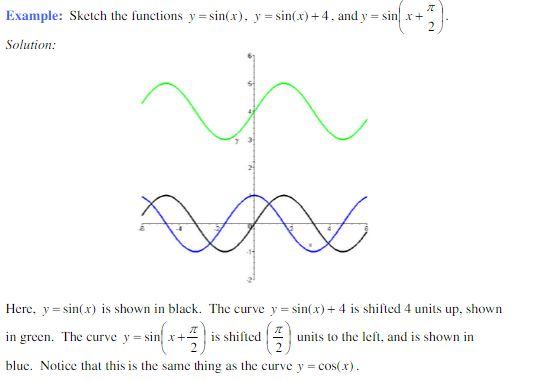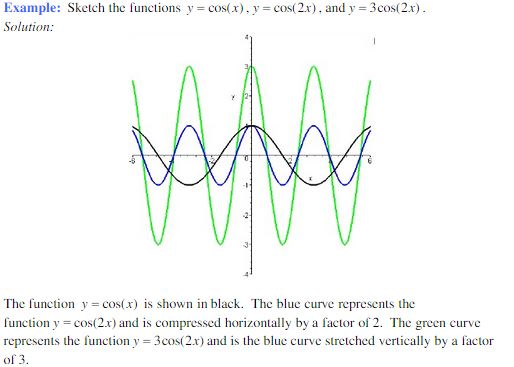Ontario Tech acknowledges the lands and people of the Mississaugas of Scugog Island First Nation.

We are thankful to be welcome on these lands in friendship. The lands we are situated on are covered by the Williams Treaties and are the traditional territory of the Mississaugas, a branch of the greater Anishinaabeg Nation, including Algonquin, Ojibway, Odawa and Pottawatomi. These lands remain home to many Indigenous nations and peoples.

We acknowledge this land out of respect for the Indigenous nations who have cared for Turtle Island, also called North America, from before the arrival of settler peoples until this day. Most importantly, we acknowledge that the history of these lands has been tainted by poor treatment and a lack of friendship with the First Nations who call them home.

This history is something we are all affected by because we are all treaty people in Canada. We all have a shared history to reflect on, and each of us is affected by this history in different ways. Our past defines our present, but if we move forward as friends and allies, then it does not have to define our future.

# Transformations of Trigonometric Functions

The transpformation of functions includes the shifting, stretching, and reflecting of their graph. The same rules apply when transforming trigonometric functions.

## Vertical and Horizontal Shifts

Suppose c > 0. To obtain the graph of

y = f(x) + c: Shift the graph of y = f(x) up by c units

y = f(x) - c: Shift the graph of y = f(x) down by c units

y = f(x - c): Shift the graph of y = f(x) to the right by c units

y = f(x + c): Shift the graph of y = f(x) to the left by c units## Vertical and Horizontal Stretches/Compressions

Suppose c > 1. To obtain the graph of

y = cf(x): stretch the graph of y = f(x) vertically by a factor of c

y = 1/c f(x): compress the graph of y = f(x) vertically by a factor of c

y = f(cx): compress the graph of y = f(x) horizontally by a factor of c

y = f(x/c): stretch the graph of y = f(x) horizontally by a factor of c## Reflections

To obtain the graph of

y = -f(x): reflect the graph of y = f(x) about the x-axis; and

y = f(-x): reflect the graph of y = f(x) about the y-axisExample 1:

Example 2: